In the last chapter Python bitwise operators", we learned python bitwise operators, their types, and their usage in Python. Python bitwise operators work on the bit level. Even though you may have two operands to be considered, they would work bit by bit to produce the desired result. Additionally, Python boolean operators are similar to python bitwise operators in the sense that instead of bits here, we consider complete boolean expressions. In Python boolean operator calculations, we make use of the boolean expressions and decide the outcome of the expressions according to the operator. Subsequently, in this tutorial, we will cover the following python boolean operators:

• What are Boolean Expressions and Boolean Operators?
• AND boolean operator in Python
• NOT boolean operator in Python
• OR boolean operator in Python

## What are the Boolean Expression and Boolean Operators?

A boolean expression is an expression that yields just the two outcomes: true or false. When we work with multiple boolean expressions or perform some action on them, we make use of the boolean operators. Since the boolean expression reveals true or false, the operations on these expressions also result in either "true" or "false". Consequently, there are three types of boolean operators:

• The AND operator (&& or "and")
• The OR operator (|| or "or")
• The NOT operator (not)

### AND Boolean Operator in Python

The AND boolean operator is similar to the bitwise AND operator where the operator analyzes the expressions written on both sides and returns the output.

• True and True = True
• True and False = False
• False and True = False
• False and False = False

Subsequently, let's try to understand this concept in plain English before we jump to code. Let's say we have the following sentence: If it does not rain today AND there are no extra classes, I will play.

• In the above example there are two expressions on which the results depend: If it does not rain today, there are no extra classes.
• To play i.e. to get the outcome as True, you need to have both the conditions true here.

This is exactly what the AND operator does except that the expressions are conditions. For example, If (a < 30 and b > 45)

In python, you can directly use the word "and " instead of "&&" to denote the "and " boolean operator while for other languages, you need to put "&&" instead. Consequently, let's execute the following code to check the output:

``````a = 30

b = 45

if(a > 30 and b == 45):

print("True")

else:

print("False")

``````

Executing the above code, we get: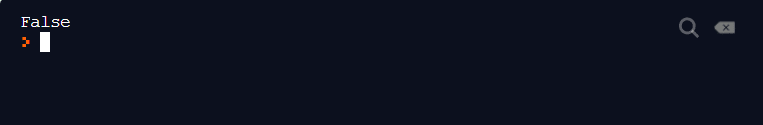Similarly, you can try random expressions by yourself to check the answer onto the console.

### OR Boolean Operator in Python

The OR operator is similar to the OR bitwise operator. In the bitwise OR, we were focussing on either of the bit being 1. Here, we take into account if either of the expression is true or not. If at least one expression is true, consequently, the result is true.

• True or True = True
• True or False = True
• False or True = True
• False or False = False

For example, taking the same example as before with "or" boolean operator, If it rains today OR there is no extra class, I will play.

• Now the conditions are the same but the situation has changed. The introduction of OR implies that if either it rains or there is no extra class or both, I will play.
• I will not play only when it does not rain today and there is an extra class i.e. both the conditions become false.

In the program, we use conditional expressions with the operator. For example, if( a > 30 || b < 45).

In python, you can use || as well as the word "or " directly into the code. Let's execute the following code to check the output:

``````a = 25

b = 30

if(a > 30 or b < 45):

print("True")

else:

print("False")
``````

Consequently, run the above code to see the result:### NOT Boolean Operator in Python

The NOT operator reverses the result of the boolean expression that follows the operator. It is important to note that the NOT operator will only reverse the final result of the expression that immediately follows. Moreover, the NOT operator is denoted by the keyword "not".

• not(True) = False
• not(False) = True

Let's try to understand/ comprehend it with the help of an example.

• If I initialize a = 30 and  b = 30, then not((a == b) will first evaluate (a == b) and then reverse the final outcome i.e. false.
• If I write the expression as not(a == b) && (c == d), here, first (a == b) is calculated. Then the answer is reversed due to the NOT operator. Then (c == d) is evaluated. Finally the AND operator will work.

Subsequently, execute the following code to run the same example we discussed in this section:

``````a = 2

b = 2

if(not(a == b)):
print("If Executed")

else:
print("Else Executed")
``````

Press Run to see the output:Since a== b is true, not(a == b) will return false.

Let's change the "if " expression now to see the executed output again:

``````a = 2
b = 2
c = 4
d = 4
if(not(a == b) and (c == d)):
print("If Executed")
else:
print("Else Executed")
``````

Execute the above code to check if else gets executed or not: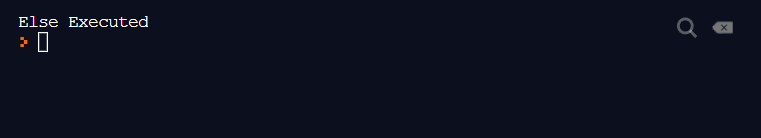If you break it down you will see that if(not(a == b) and (c == d)) gets evaluated to

if (not(true) and true) gets evaluated to if*( false and true)* which results in if (false) - That's the reason you will see that if doesn't get executed and you see "Else Executed " printed on the console.

Now that we have learned about these operators, let's see how they help us simply the code. Additionally, the following code prints out the greatest number among three numbers with the help of if-else and without the use of operators:

``````a = 10
b = 30
c = 20
if( a > b):
if(a > c):
print("a")
else:
print("c")
elif(b > c):
print("b")
else:
print("c")
``````

Executing the above code brings about the following output in the console: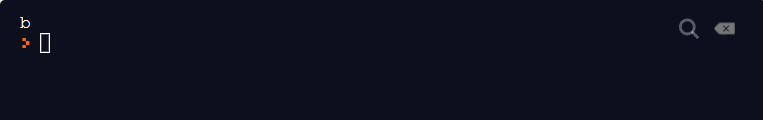With what we have learned so far, can you try to optimize the code using the boolean operator? Try it before looking at the solution below.

``````a = 10
b = 30
c = 20
if( a > b and a > c):
print("a")
elif(b > a and b > c):
print("b")
else:
print("c")
``````

We have converted the above logic of 9 lines into a logic of 6 lines using boolean operators. A similar effect can be seen in hundreds of lines of code.

This brings us to the end of the boolean operators. These operators are important not only in Python but in any programming language as it helps us to build logic into the program. Knowing the precedence and execution flow of these operators is extremely necessary because such errors are not highlighted by the compiler. So, if you find something wrong with the results, there are great chances that at some point any operator got misplaced. Keep practicing with different use cases to gain mastery over the operators.

## Key Takeaways:

• Firstly, Boolean operators are used in a boolean expression to return boolean values.
• Secondly, Boolean operators can compress multiple if-else boolean expressions into one single line of code.
• Lastly, there are three types of python boolean operators:
• AND operator
• OR operator
• NOT operator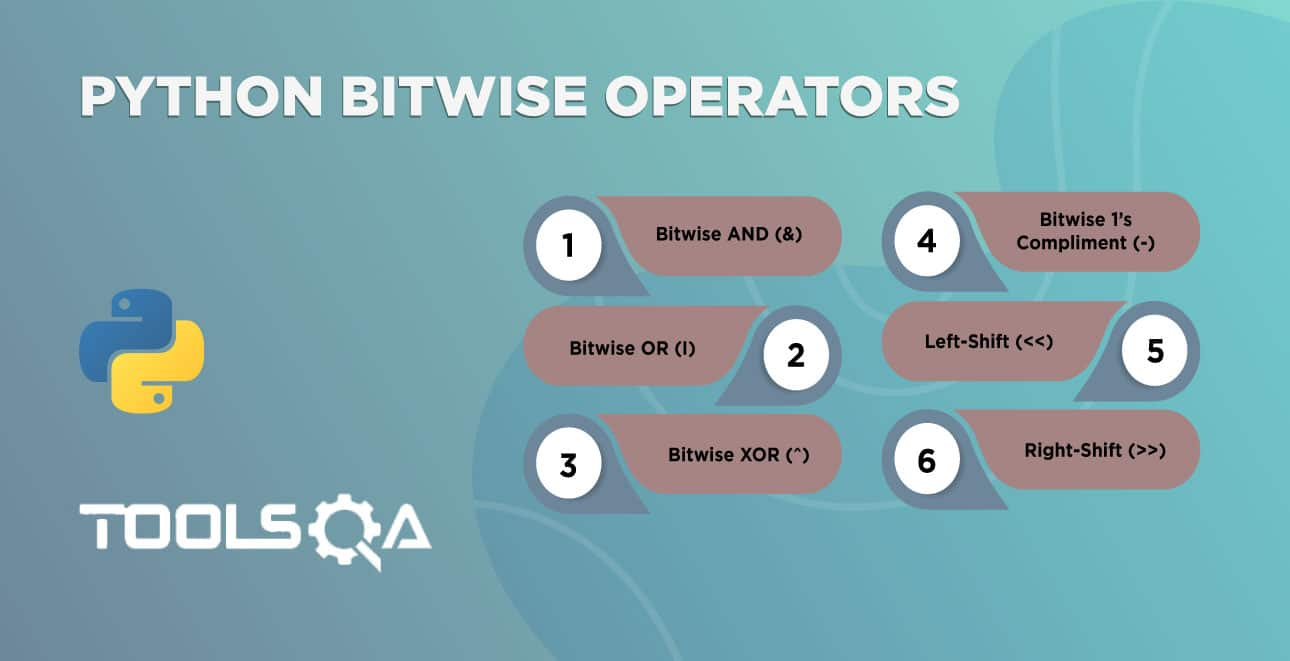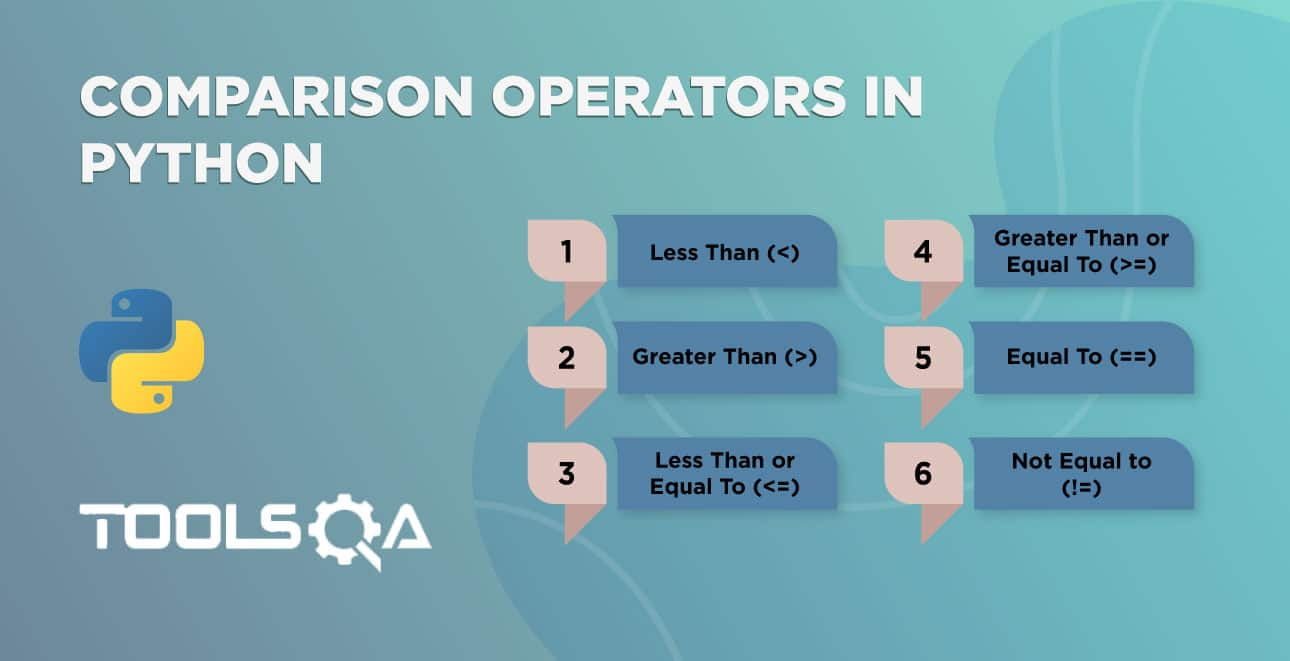ReviewersLakshay Sharma